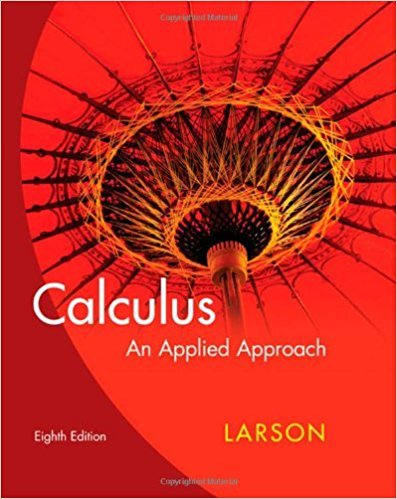×
×

# Solutions for Chapter 2.7: Implicit Differentiation## Full solutions for Calculus: An Applied Approach | 8th Edition

ISBN: 9780618958252Solutions for Chapter 2.7: Implicit Differentiation

Solutions for Chapter 2.7
4 5 0 265 Reviews
22
0
##### ISBN: 9780618958252

Since 47 problems in chapter 2.7: Implicit Differentiation have been answered, more than 24094 students have viewed full step-by-step solutions from this chapter. This expansive textbook survival guide covers the following chapters and their solutions. This textbook survival guide was created for the textbook: Calculus: An Applied Approach , edition: 8. Calculus: An Applied Approach was written by and is associated to the ISBN: 9780618958252. Chapter 2.7: Implicit Differentiation includes 47 full step-by-step solutions.

Key Calculus Terms and definitions covered in this textbook
• Angle

Union of two rays with a common endpoint (the vertex). The beginning ray (the initial side) can be rotated about its endpoint to obtain the final position (the terminal side)

• Compounded monthly

See Compounded k times per year.

• Determinant

A number that is associated with a square matrix

• Double-blind experiment

A blind experiment in which the researcher gathering data from the subjects is not told which subjects have received which treatment

• Event

A subset of a sample space.

• Factor

In algebra, a quantity being multiplied in a product. In statistics, a potential explanatory variable under study in an experiment, .

• Graph of a function ƒ

The set of all points in the coordinate plane corresponding to the pairs (x, ƒ(x)) for x in the domain of ƒ.

• Inverse cosine function

The function y = cos-1 x

• Linear inequality in x

An inequality that can be written in the form ax + b < 0 ,ax + b … 0 , ax + b > 0, or ax + b Ú 0, where a and b are real numbers and a Z 0

• Linear system

A system of linear equations

• Matrix element

Any of the real numbers in a matrix

• Open interval

An interval that does not include its endpoints.

• Ordered pair

A pair of real numbers (x, y), p. 12.

• Parametric equations for a line in space

The line through P0(x 0, y0, z 0) in the direction of the nonzero vector v = <a, b, c> has parametric equations x = x 0 + at, y = y 0 + bt, z = z0 + ct.

• Product of matrices A and B

The matrix in which each entry is obtained by multiplying the entries of a row of A by the corresponding entries of a column of B and then adding

• Reference triangle

For an angle ? in standard position, a reference triangle is a triangle formed by the terminal side of angle ?, the x-axis, and a perpendicular dropped from a point on the terminal side to the x-axis. The angle in a reference triangle at the origin is the reference angle

• Simple harmonic motion

Motion described by d = a sin wt or d = a cos wt

• System

A set of equations or inequalities.

• Vertical line test

A test for determining whether a graph is a function.

×